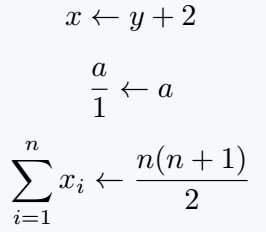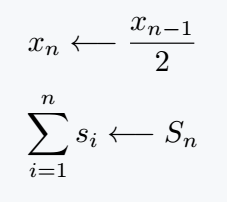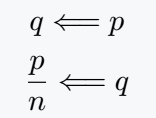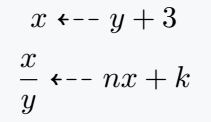# How to use left arrows in LaTeX?

Left arrows are commonly used in various mathematical and graphical contexts to indicate a direction or relation towards the left. LaTeX provides us with several symbols and commands to insert and use different types of left arrows.

In this tutorial, we will explore how to insert and utilize various left arrow symbols in LaTeX.

## Inserting Left Arrow Symbols

To insert a left arrow symbol in LaTeX, you can use the corresponding command within math mode or text mode. For example, to insert a left arrow symbol in an equation, place the command in mathmode.

Properties Value
Symbol Left arrow symbol
Argument No
Command \leftarrow
Example x \leftarrow y## Left simple arrow

This symbol is commonly used in assignments or equations to represent variable assignment or updating a value. LaTeX command for this symbol is \leftarrow always placed in mathmode

\documentclass{article}
\begin{document}
$x \leftarrow y + 2$
$\frac{a}{1} \leftarrow a$
$\sum_{i=1}^{n} x_i \leftarrow \frac{n(n+1)}{2}$
\end{document}


Output :## Long Left Arrow

Long left arrow symbol is often used in mathematical algorithms or sequences to indicate a step or process. LaTeX command for this symbol is \longleftarrow always placed in mathmode.

\documentclass{article}
\begin{document}
$x_n \longleftarrow \frac{x_{n-1}}{2}$
$\sum_{i=1}^n s_i \longleftarrow S_n$
\end{document}


Output :## Double Leftwards Arrow

Double leftwards arrow symbol is used to indicate that a statement is implied or follows from another statement. To insert this symbol, use the command \Longleftarrow within mathmode.

\documentclass{article}
\begin{document}
$q \Longleftarrow p$
$\frac{p}{n} \Longleftarrow q$
\end{document}


Output :## Leftwards Dashed Arrow

Leftwards dashed arrow symbol is commonly used in mathematical expressions to indicate a tentative or provisional step. To insert this symbol, use the command \dashleftarrow within mathmode.

But, leftwards dashed symbol default is not present. For this, you need to use external package amsfonts or amssymb.

\documentclass{article}
\usepackage{amssymb}
\begin{document}
$x \dashleftarrow y + 3$
$\frac{x}{y} \dashleftarrow nx + k$
\end{document}


Output :These examples demonstrate the usage of this symbol in mathematical contexts, showcasing their specific meanings and applications.

Below is a list the most common types of left arrow you might likely come across with their LaTeX Commands

Arrow Name LaTeX Command
Leftwards Arrow \leftarrow
Long Left Arrow \longleftarrow
Double Left Arrow \Leftarrow
Long Leftwards Arrow \Longleftarrow
Leftwards Arrow Over Rightwards Arrow \leftrightarrows
Leftwards Dashed Arrow \dashleftarrow

These examples demonstrate how each left arrow symbol can be used in mathematical contexts to express various relationships, transformations, implications, or sequences.

Specific symbol used depends on the intended meaning and context within the mathematical expression or equation.

These symbols can be used in combination with other LaTeX commands and environments to express various directional relationships and operations.

## Conclusion

LaTeX provides a set of symbols and commands for inserting and using left arrow symbols in various mathematical and textual contexts.

By leveraging these symbols and combining them with other LaTeX commands and environments, you can express directional relationships and logical implications effectively.

Remember to use appropriate math mode delimiters or dollar signs to incorporate left arrow symbols within equations and text.#### Md Jidan Mondal

LaTeX expert with over 10 years of experience in document preparation and typesetting. Specializes in creating professional documents, reports, and presentations using LaTeX.